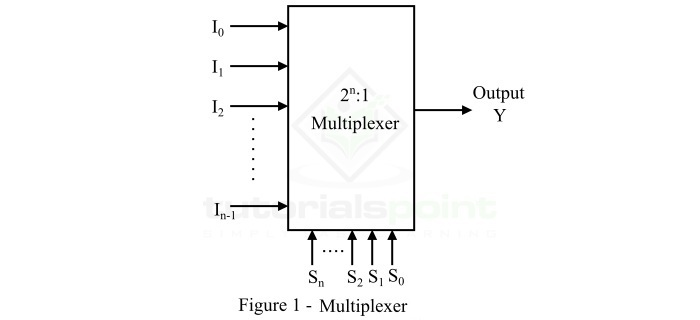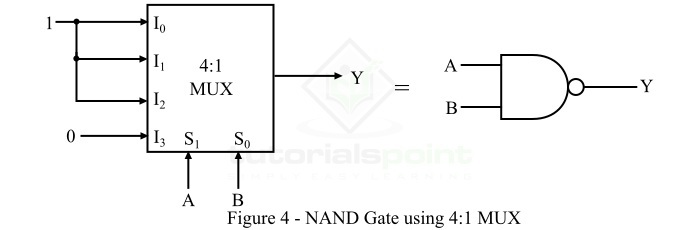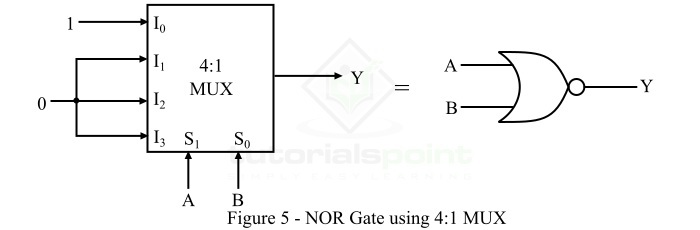# Multiplexer as Universal Logic Gate

Read this article to learn how you can use a multiplexer to realize universal logic gates. Let us start with a brief introduction to multiplexers and universal logic gates.

## What is a Multiplexer?

A Multiplexer or MUX or Data Selector is a combinational logic circuit which accepts several data inputs and allows only one of them at a time to pass through the output channel. The block diagram of a multiplexer is shown in Figure-1.A multiplexer consists 2n data input lines, n select lines, and only one output line. The logic level applied to the select lines determine which input will pass to the output channel.

Depending on the number of data input lines, multiplexers can be of several types such as 2:1 MUX, 4:1 MUX, 8:1 MUX, etc. In digital electronics, multiplexers are used in several application like realization of Boolean functions and different types of logic gates.

## What is a Universal Gate?

A Universal Logic Gate is a type of logic gate (a digital device used to perform a logical operation) which can be used to implement any kind of logic function or other basic logic gates such as OR, AND, NOT, etc.

In digital electronics, we have two universal logic gates namely, NAND Gate and NOR Gate.

### NAND Gate

The NAND Gate is a type of universal logic gate. The NAND gate is basically a combination of two basic logic gates namely AND gate and NOT gate, i.e.,

NAND Logic = AND Logic + NOT Logic


The NAND gate is the logic gate whose output is LOW when all its inputs are high, and its output is HIGH, when any of its inputs is LOW. Therefore, the operation of the NAND gate is opposite that of the AND gate. The logic symbol of a two input NAND gate is shown in Figure-2.For the NAND gate, if A and B are the input variables and Y is the output variable, then its output equation is given by,

$$Y=\overline{A\cdot B}=\left ( A\cdot B \right )'$$

It is read as “Y is equal to A.B whole bar”.

The operation of the NAND gate for different possible combinations of inputs can be analyzed with the help its truth table which is given below −

Inputs Output
A B Y = (A.B)'
0 0 1
0 1 1
1 0 1
1 1 0

### NOR Gate

The NOR Gate is another type of universal logic gate. Here, NOR means NOT + OR. That means, the OR output is NOTed or inverted. Thus, the NOR gate is a combination of OR gate and a NOT gate, i.e.,

NOR Gate = OR Gate + NOT Gate


The NOR gate is a logic gate whose output is HIGH, only when all its inputs are LOW, and it gives an output LOW, even if any of its inputs becomes HIGH. The logic symbol of a two input NOR gate is shown in Figure-3.For a NOR gate, if A and B are the input variables and Y is the output variable, then the output equation of the NOR gate is given by,

$$Y=\overline{A + B}=\left ( A + B \right )'$$

It is read as “Y is equal to A plus B whole bar”.

We can analyze the operation of the NOR gate for different possible combinations of inputs by its truth table which is given below −

Inputs Output
A B Y = (A+B)'
0 0 1
0 1 0
1 0 0
1 1 0

Now, after getting a brief description about multiplexer, NAND gate and NOR gate. We can discuss the implementation of these universal logic gates using multiplexer.

## NAND Gate using Multiplexer

The implementation of a two input NAND gate using multiplexer is shown in Figure-4.Since a two input NAND gate can have total four (22 = 4) possible combinations of input variables. Hence, to realize a two input NAND gate, we require a 4:1 MUX.

According the truth table of two input NAND gate, the output Y = 1 for first three combinations, i.e. 00, 01, 10. Thus, the input lines I0, I1, and I2 of the multiplexer are connected to logic 1. The output of the NAND gate Y = 0 for combination 11, thus, the input line I3 of MUX is connected to logic 0.

In this way, we can realize the NAND gate using a multiplexer. Now, let us discuss the implementation of NOR gate using multiplexer.

## NOR Gate using Multiplexer

The implementation of a two input NOR gate using a multiplexer is shown in Figure-5.Since a two input NOR gate can have total four (22 = 4) possible combinations of input variables. Hence, to realize a two input NOR gate, we require a 4:1 MUX.

The input variables A and B of the NOR gate are used as the select lines of the multiplexer. Where, A and B are applied to S1 and S0 respectively.

As per the truth table of the two input NOR gate, the output Y = 1 for only first combination of input variables, i.e. 00. Hence, we apply logic 1 only to the data input line I0 of the multiplexer. The output of the NOR gate Y = 0 for all other combinations, i.e. 01, 10, and 11, therefore, the input lines I1, I2, and I3 of the MUX are connected to logic 0. This way, a NOR gate can be realized using a multiplexer.

## Tutorial Problems

Try to solve the following tutorial problems to excel in the concept of using a multiplexer to realize universal logic gates.

Q. 1 − Implement a three input NAND gate using an 8:1 MUX.

Q. 2 − Implement a three variable NOR gate by using an 8:1 MUX.

Updated on: 19-Apr-2023

903 Views